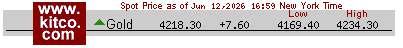# What is my gold worth?

We all want to know the value of our gold items before we sell them so we do not get ripped off by our local gold buyer. The easiest way to determine the value of your gold is to use our precious metals calculator or our free mobile app.

If you want to know the technical way to determine the value of your precious metals then keep reading this page.  Start by separating your gold into individual piles of each Karat value like 10k, 14k, 18k etc. The next step is to calculate the weight of all your gold items using an accurate scale.Scales weigh in grams, ounces, troy ounces or pennyweight. It is recommended that your scale weighs in smaller increments or multiple decimal places.

Here is a quick tip to make sure your scale is calibrated properly. United States coins such as pennies, nickels, quarters, and dimes have exact weight specifications which allow you to weigh coins individually or in groups to verify how accurate your scale is. For example a quarter weighs 5.670 grams.

If you do not have a scale you can use the above coin weight method to guess the weight of your items. For example: My items weigh close to the same as three quarters and one penny. This method will not give you an exact weight but it can give you a good indication of how much gold you might have.

Let's explore the different types of weights your scale can measure. There is 31.1 grams in a troy ounce (ozt) and there are 28 grams in an ordinary ounce (oz). Precious metals like gold are typically weighed in troy ounces. Food and drugs are weighed by ordinary ounces. . There are 20 pennyweight (dwt) per troy ounce. Example of conversion: 20 pennyweight multiplied by 1.556 = 31.1 grams.

You can google precious metal calculators which will do all the easy math for you and can determine the actual value of your items. You will just need to enter the spot value of gold along with the weight of your items and the actual karat of gold. Most precious metal calculators will determine the true value of your gold but some will actually reduce it by a specific percentage since that is what they are willing to pay for your gold.

Click to use our Precious metal Calculator

This example will calculate 1 gram of 14k gold when the gold spot price is \$1700.00 per troy ounce.

Divide 14 (14k item) by 24 (24K is pure gold) and it should equal .583 which is equal to 58.3 % pure gold content.

Next take the spot price of \$1700.00 and divide that by 31.1 which is the amount of grams per troy ounce to determine that 1 gram of pure 24k gold equals \$54.66 then multiply x the percentage of gold content of .583 and we determine that 1 gram is worth \$31.87

If you have many grams of 14k we just multiply the \$31.87 x the amount of grams. This is the true market value of your gold.

So the simple formula is \$1700.00 divided by 31.1 x .583 x 1 gram = \$31.87

Use the Kitco gold value indicator which is the current spot price of gold. This value is bought and sold on the open market and can go up or down by the minute just like any ordinary stock on the stock exchange. 2011 through 2013 have been historic years for gold value and there has never been a better time than the present to sell your gold.